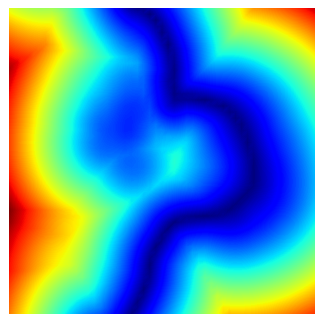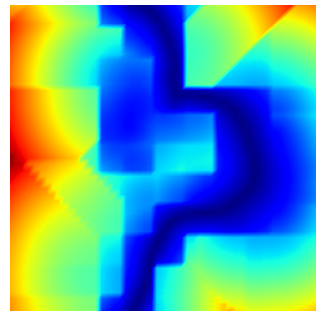# vtkStaticCellLocator problem (vtk9.0.3)

I observe something strange in `vtkStaticCellLocator.FindClosestPoint()` vs `vtkCellLocator.FindClosestPoint()`:

with vtkStaticCellLocator (wrong):

with vtkCellLocator (correct):

``````from vedo import *

# Load and cut mouse limb mesh
limb = Mesh("https://vedo.embl.es/examples/data/270_flank.vtk")
limb.alpha(0.3).c("purple9").lighting("off")

# Make a wall in the cut limb to show gradient
grid = Grid(sx=2000, sy=2000, resx=50, resy=50).rotateX(90)
grid.cutWithMesh(limb).wireframe(False)

# scaling things makes no difference
#limb.scale(0.001)
#grid.scale(0.001)

values = []
for p in grid.points():
q = limb.closestPoint(p)
values.append(mag(p-q))

show(limb, grid, viewup='z', axes=1)
``````

The range is also very different.
The name of the two classes is literally the only thing I change between the two… Any ideas?

This seems to be a bug. What happens if you run the same script but replace `vtkStaticCellLocator` by `vtkStaticPointLocator`?

The behavior of the two classes should be the same. Could you create an equivalent VTK python script…

…unless i’m doing something silly…:

``````import vtk
from vedo import Mesh, Grid, mag, show

# load the data as VTK objs
limb = Mesh("https://vedo.embl.es/examples/data/270_flank.vtk")
lpoints = limb.points()  # numpy array
limb = limb.polydata()   # vtkPolyData
grid = Grid(sx=2000, sy=2000, resx=50, resy=50)
gpoints = grid.points()  # numpy array
grid = grid.polydata()   # vtkPolyData

locator1 = vtk.vtkCellLocator()
locator1.SetDataSet(limb)
locator1.BuildLocator()

locator2 = vtk.vtkStaticCellLocator()
locator2.SetDataSet(limb)
locator2.BuildLocator()

locator3 = vtk.vtkPointLocator()
locator3.SetDataSet(limb)
locator3.BuildLocator()

locator4 = vtk.vtkStaticPointLocator()
locator4.SetDataSet(limb)
locator4.BuildLocator()

values1 = []
values2 = []
values3 = []
values4 = []
for p in gpoints:
q = [0, 0, 0]
cid = vtk.mutable(0)
dist2 = vtk.mutable(0)
subid = vtk.mutable(0)
locator1.FindClosestPoint(p, q, cid, subid, dist2)
values1.append(mag(p-q))

q = [0, 0, 0]
cid = vtk.mutable(0)
dist2 = vtk.mutable(0)
subid = vtk.mutable(0)
locator2.FindClosestPoint(p, q, cid, subid, dist2)
values2.append(mag(p-q))

id3 = locator3.FindClosestPoint(p)
values3.append(mag(p-lpoints[id3]))

id4 = locator4.FindClosestPoint(p)
values4.append(mag(p-lpoints[id3]))

print(max(values1), max(values2)) # should be the same
print(max(values3), max(values4)) # should be the same (and they are)

# show(Mesh(grid).cmap("jet", values2))
``````

outputs:

``````795.8835103198636 993.0246205222754
795.8835103198636 795.8835103198636
``````I just run this script in ParaView master and I do get the correct max values. What version of VTK are you using?

it’s
vtk version : 9.0.3
python version : 3.8.8 (default, Apr 13 2021, 19:58:26) [GCC 7.3.0]
python interpreter: /home/musy/soft/anaconda3/bin/python
system : Linux 5.4.0-99-generic posix x86_64

There was a bug fix made to vtkStaticCellLocator related to FindClosestPoint (commit 2b07d64ea made about a year ago…)

1 Like

Yep, you should update to VTK 9.1.0 to have this fixed.

1 Like